Graph IDEData Graphics ► Function
(a.k.a.: Curve or Line Graph)

A function is a sequence of points connected by line segments. The x-values of the points increase as the sequence index increases. The figures below show examples of functions.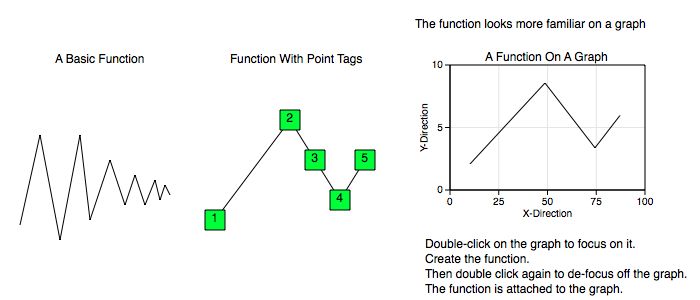All Data Graphics, including this function graphic, transform according to the coordinates of the graph it is on. So, for example, the following graphs show the same exact function graphic (and same x and y point data) but on two different coordinate types. In the polar graph, the radius is mapped with an absolute value function. For additional information consult the Non-Linear Graphs section.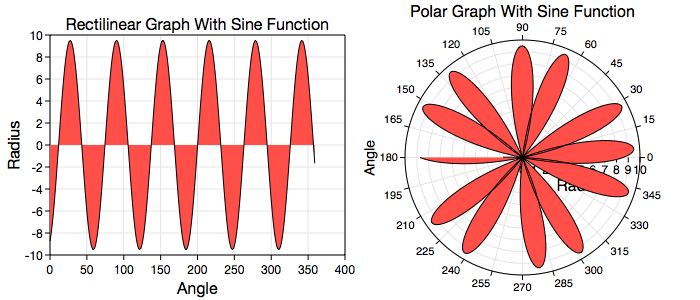Some standard operations are itemized below.

• To create a function bring forward the Graphic Selector, click the function factory cell and then mouse down on a Graphic View and drag the cursor to another point. The initial and final cursor locations define the boundary of a predefined function graphic.

• You can also create functions from the standard Palettes2D GraphsSix Functions menu item as well as other palettes or drag them out from the function Factory Inspector.

• Resizing the function transforms all points simultaneously. A function cannot be rotated because it has to maintain its function properties. For more information on editing a function, including point-wise editing, see Standard Editing.

• Once a function is created, the usual way to modify its data values is through the Table on its inspector editor.

• To program a function see the Programming section.

Data Editor

The Data Editor for the Function is shown below.Function Specific Controls

Operations : Select this to perform common sequence operations upon the data. The most important sequence operation is Sort X-Ascending because the table data should always be x-ascending.

Table

Table controls are described in the Tables section.

The rows represent vertex values. While in Atomic mode, the cell represents a point (x and y value) and while in component mode the cell represents either a x or y value. Hence, in atomic mode there is one column while in component mode there are two columns.

If points are imported into the table or edited and that operation does not produce x-ascending data then the table should be resorted using the Operations control, i.e.: x-ascending is not enforced and needs to be done explicitly.

X-ascending data is a convention that helps with optimization. It is also required to meet the definition of the Function graphic.

Graphics Editor

The Graphics Editor for the Function is shown below.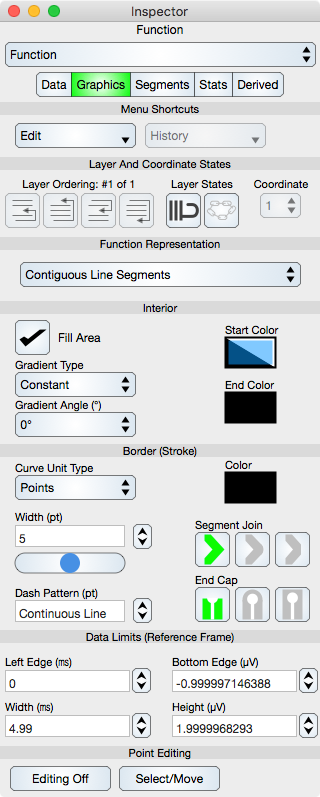Common Controls

Controls common to all graphics are described in the Graphics section.

Point Editing

Editing Off/On : Places the graphic into or out of edit mode. While in edit mode the vertices are shown by indicators and can be adjusted using mouse or touch events. Double-clicking the graphic also toggles this edit mode.

Select/Move or Add/Delete : Select/Move mode permits the vertex editing to select and move those locations while Add/Delete mode will delete a vertex if it is hit or add a vertex if a Function segment is hit. This can also be accomplished using the shift key if available.

Segments Editor

The Segments Editor for the Function is shown below.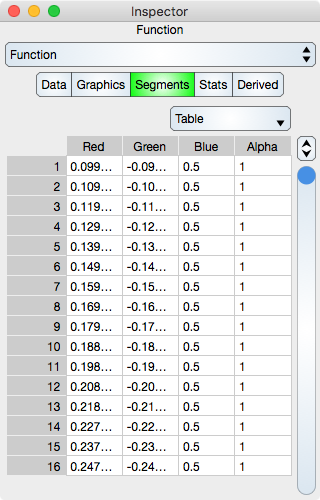Segments are the line segments between adjacent points. See Sequence Colors for additional information.

Table

Table controls are described in the Tables section.

The rows represent segment color values. While in Atomic mode, the cell represents a rgba value and while in component mode the cell represents either single r, g, b and a. Hence, in atomic mode there is one column while in component mode there are four columns.

Note that if the Data sequence is resorted then the Segments sequence will not be resorted.

Stats Editor

The Stats Editor provides basic statistics for the Function and is shown below.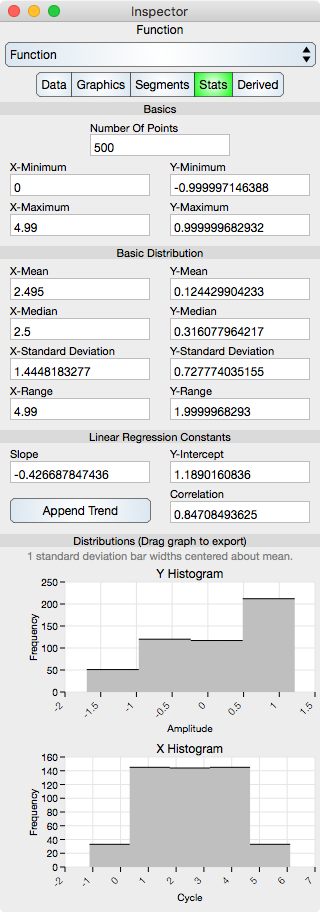Basics

Number Of Points : Shows the number of points in the Function.

X-Minimum : x-minimum value of all the data points. Since the data points should be x-ascending, the x-minimum is the x-value of the first data point in the sequence.

Y-Minimum : y-minimum value of all the data points.

X-Maximum : x-maximum value of all the data points. Since the data points should be x-ascending, the x-maximum is the x-value of the last data point in the sequence.

Y-Maximum : y-maximum value of all the data points.

Basic Distribution

The basic distribution is shown separately for the x-dimension and the y-dimension. For a function, the y-distribution is the important distribution.

Mean : Shows the mean of the data.

Median : Shows the median of the data.

Standard Deviation : Shows the standard deviation of the data.

Range : Shows the range of the data (maximum - minimum).

Linear Regression Constants

Note that linear regression may also be referred to as line fit.

Slope : Shows the slope of the linear regression.

Y-Intercept : Shows the y-intercept of the linear regression.

Correlation : Shows the correlation constant of the linear regression.

Distributions

Y Histogram : Shows the y-histogram of the data. This graph can be dragged onto the Graphic View.

X Histogram : Shows the x-histogram of the data. This graph can be dragged onto the Graphic View.

© Copyright 1993-2019 by VVimaging, Inc. (VVI); All Rights Reserved. Please email support@vvi.com with any comments you have concerning this documentation. See Legal for trademark and legal information.